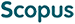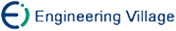周树民, 李锦涛, 黄 晁, 宋 磊. 混合视频编码控制中一种新的自适应Lagrange乘数选取模型[J]. 计算机研究与发展, 2005, 42(11): 1876-1881.
 引用本文: 周树民, 李锦涛, 黄 晁, 宋 磊. 混合视频编码控制中一种新的自适应Lagrange乘数选取模型[J]. 计算机研究与发展, 2005, 42(11): 1876-1881.Zhou Shumin, Li Jintao, Huang Chao, Song Lei. A New Adaptive Lagrange Multiplier Selection Model in Hybrid Video Coder Control[J]. Journal of Computer Research and Development, 2005, 42(11): 1876-1881.
 Citation: Zhou Shumin, Li Jintao, Huang Chao, Song Lei. A New Adaptive Lagrange Multiplier Selection Model in Hybrid Video Coder Control[J]. Journal of Computer Research and Development, 2005, 42(11): 1876-1881.## A New Adaptive Lagrange Multiplier Selection Model in Hybrid Video Coder Control

• 摘要: 目前广为采用的Lagrange乘数选取模型λ=cQ2(平方模型SM)是在基于乘数λ为独立于失真D和比特率R的常数这一假定而得出的.但实际上根据实验观察和理论分析可知，相对于D和R乘数λ不能看做是独立的常数.基于这一命题，从理论推理的角度提出了新的乘数模型(二次函数-对数模型QLM).该模型相对于平方模型，理论上更为合理，而且实验分析也表明，采用该模型后R-D性能得到了提高(平均提高0.03dB)，从而证明了所提模型的合理性.

Abstract: The equation λ=cQ2 has been widely used as a parameter selection model in hybrid video coder control with Lagrange rate-distortion optimization, which is called square model (SM) and deduced with an assumption of λ being constant. The coefficient c is obtained from statistic in different video coding recommendations. However, according to the theoretical analysis, λ shouldn't be seen as a constant. A proposition about this idea is proposed and proved by mathematically deducing and experimental justification. Based on the proposition, a new Lagrange multiplier selection model called quadratic-logarithm model (QLM) is deduced and its parameter is adaptively obtained by the scheme proposed in the paper. Finally, QLM is used in H.264 and a new rate-distortion scheme is proposed. The final simulation result shows that compared with SM, the new multiplier selection model can obtain better rate-distortion performance in general sense (0.03dB ave.) without any further extra computation. Therefore, the multiplier model proposed is more feasible and reasonable than the existing ones./下载:  全尺寸图片 幻灯片
• 分享
• 用微信扫码二维码

分享至好友和朋友圈